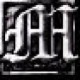Feeds:
Posts

## The Lawvere theory of Boolean functions

Let$2$ be a set with two elements. The category of Boolean functions is the category whose objects are the finite powers$2^k, k \in \mathbb{Z}_{\ge 0}$ of$2$ and whose morphisms are all functions between these sets. For a computer scientist, the morphisms of this category have the interpretation of functions which input and output finite sequences of bits.

Since this category has finite products and is freely generated under finite products by a single object, namely$2$, it is a Lawvere theory.

Question: What are models of this Lawvere theory?

The answer is that they are Boolean algebras, and also that they are Boolean rings. Equivalently, the standard axiomatizations of Boolean algebras resp. Boolean rings describe two different presentations of the Lawvere theory of Boolean functions. In the Boolean algebra presentation, the generators are

• two constants (true and false, or$1$ and$0$),
• a unary operator$\neg$ (NOT), and
• two binary operators$\wedge, \vee$ (AND and OR),

while in the Boolean ring presentation, the generators are

• two constants ($1$ and$0$),
• two binary operators$\times, +$ (AND and XOR).

The relations are given by the various axioms that these satisfy.

It’s an interesting observation that either of these small sets of Boolean functions already suffice to build arbitrary ones. This means that logic circuits built out of these basic components can compute any Boolean function, which is maybe the zeroth interesting theorem in computer science. (The first interesting theorem, which is much more interesting, is the existence of universal Turing machines.)

Here’s a proof that either of these sets of Boolean functions generate all Boolean functions, although I won’t prove anything about relations. We only need to worry about Boolean functions of the form$f : 2^k \to 2$. By conditioning on whether or not the first bit of the input is$1$ or$0$, the data of such a Boolean function is equivalent to the data of two other Boolean functions$g_1, g_0 : 2^{k-1} \to 2$, namely the Boolean function of the other bits of the input you get if the first bit of the input is$1$, and the function you get if the first bit of the input is$0$. In terms of these two functions,$f$ itself can be described as “if the first bit is$1$, then return$g_1$; else, return$g_0$.”

Inducting on$k$, this argument shows that all Boolean functions can be generated by

• two constants ($1$ and$0$), and
• a ternary operator “if-then-else.”

This ternary operator is known in computer science as the conditional operator or ternary operator and in logic as conditional disjunction.

(I once spent some time asking myself what a programming language is from the point of view of category theory. My tentative answer is that a programming language is a tool for naming morphisms in categories. We can think about the generators above as a toy programming language whose only data type is booleans and whose only control structure is if-then-else, and the way in which I happened upon it was as a tool for naming morphisms in the Lawvere theory of Boolean functions. More complicated programming languages can be thought of as tools for naming morphisms in more complicated categories, such as cartesian closed categories.)

It follows that in order to show that some collection of Boolean functions generates all Boolean functions (a condition known in logic as functional completeness), it suffices to show that it can generate$1, 0$, and if-then-else.

In terms of logical implication$\rightarrow$, and as a function of three bits$p, q, r$, if-then-else can be written$(p \rightarrow q) \wedge (\neg p \rightarrow r)$.

Logical implication$p \rightarrow q$ can itself be written as$\neg p \vee q$, so if-then-else can be written in terms of$\neg, \vee, \wedge$ (NOT, OR, AND). This shows that the Boolean algebra generators generate all Boolean functions. To show that the Boolean ring generators also generate all Boolean functions, we need to write NOT, OR, and AND in terms of XOR ($+$) and AND ($\times$). We can get$\neg p$ using$1 + p$, and we already have AND, which means we can get OR using$\neg (\neg p \wedge \neg q) = 1 + (1 + p)(1 + q) = p + q + pq$.

Hence the Boolean ring generators also generate all Boolean functions.

### 3 Responses

1. on February 24, 2017 at 3:07 pm | ReplyMike Stay

There’s a similar Lawvere theory for any topos, right? The objects are finite powers of the subobject classifier and the morphisms are all the homomorphisms between them. Is there a name for these algebras?

In particular, take the subobject classifier for (directed multi)graphs, aka quivers; is there a presentation of this Lawvere theory with nullary, unary, and binary function symbols like the one in your post? What are the models? Since discrete graphs are the same as sets, it seems like the algebras would be special Boolean algebras.

2. […] algebra or Boolean ring (more invariantly, the structure of a model of the Lawvere theory of Boolean functions) in any distributive category. Hence any distributive category naturally admits a contravariant […]

3. on November 26, 2015 at 9:36 pm | ReplyJon Awbrey